# OFEP2: Improved RMS optics configurations

OFEP 2
Author Julian Stirling
Created 14-Nov-2020
Status Approved
Approved date 26-May-2021
Requires implementation Yes
Implemented date N/A
Updated dates (post-approval) N/A

## 1. Introduction

This OpenFlexure Enhancement Proposal relates to the stability and configurability of the optics in the OpenFlexure microscope. It will both streamline the code for producing optics modules for RMS objectives, and improve the ease of configuring the optics module for a new tube lens/objective combination.

### 1.1 Definition of terms

Much of the terminology for microscopes refers to components in a traditional (not digital) microscope. In its most basic form the imaging components of a traditional microscope consists of an objective and an eyepiece attached to a tube. Objectives are designed for a tube of a given length (the mechanical tube length or tube length), and to be a specific distance from the sample (parfocal distance). Both of these measurements are specified from the end of the tube the objective is screwed into. A shoulder on the objective sets where the objective meets the end of this tube.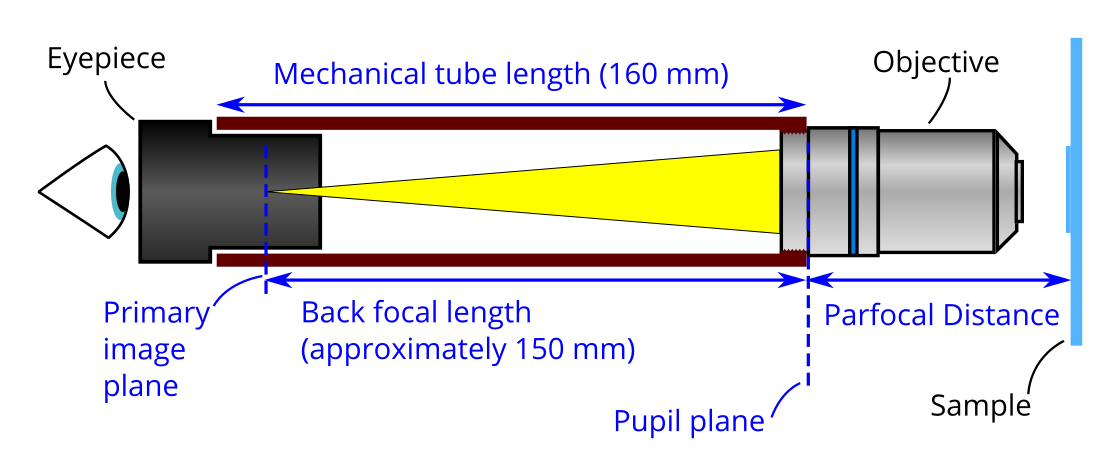Many common objectives are designed for mechanical tube length of 160 mm, and have a parfocal distance of 45 mm (35 mm is also available). Some more expensive objectives have an infinite tube length, and require an extra lens to form an image (more on this later).

Light exits the objective at the exit pupil, which lies on the pupil plane approximately in line with the shoulder. An image is formed on the primary imaging plane of the eyepiece, where it is viewed by the user. When building the OpenFlexure Microscope we use a camera sensor (a camera with the lens removed) instead of an eyepiece. We need the plane of the camera sensor to be where the primary image plane of the eyepiece would be. To reduce the size of the microscope and to increase the field of view (see section 3.3.) we use a extra lens called a tube lens.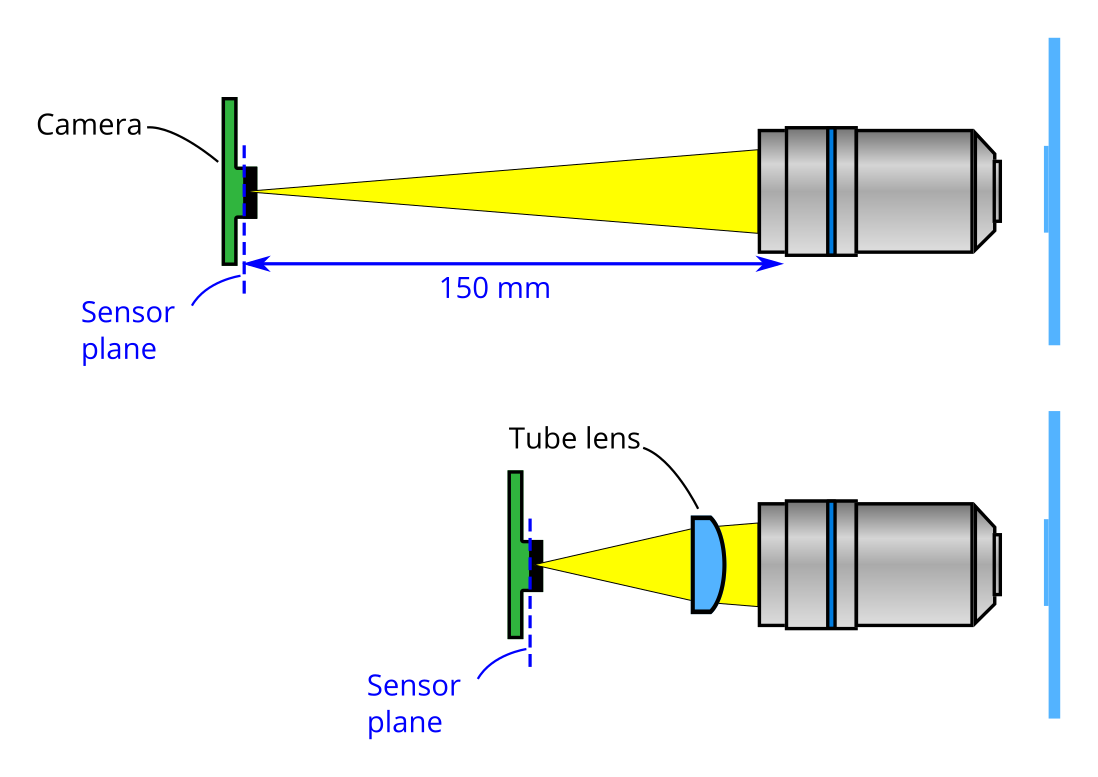The position of the camera now depends on both the position of this tube lens, and the focal distance of the lens. Using a tube lens also allows us to use an infinite conjugate objective. Light exiting an infinite conjugate objective is collimated (or is said to form an image at infinity). As such, a tube lens is always needed to form an image inside a microscope if an infinite conjugate objective.

## 2. State prior to this OFEP

Note: This section explains how the RMS optics were configured in v6.1.5. Section 3. explains how optics will be configured after this OFEP

The standard configuration OpenFlexure RMS optics modules perform very well, with the most tested version being the module for a finite conjugate objective and a 50 mm focal length tube lens. However, there are a numerous versions that are less regularly checked which we our build script generates as standard, but may not work. Also due to legacy code the optics modules are very difficult to modify.

Currently the position of the objective and tube lens are set by the sample position where as the camera position is set based off the bottom of the microscope. The camera position is precalculated for 3 tube-lens/objective configurations. As such:

• It is hard to use a custom tube lens as the documentation on calculating camera position is sparse.
• The optics modules get stretched if the microscope stage is height is changed.

### 2.1. Geometry

The current geometrical parameters are explained below (as they were in v6.1.5):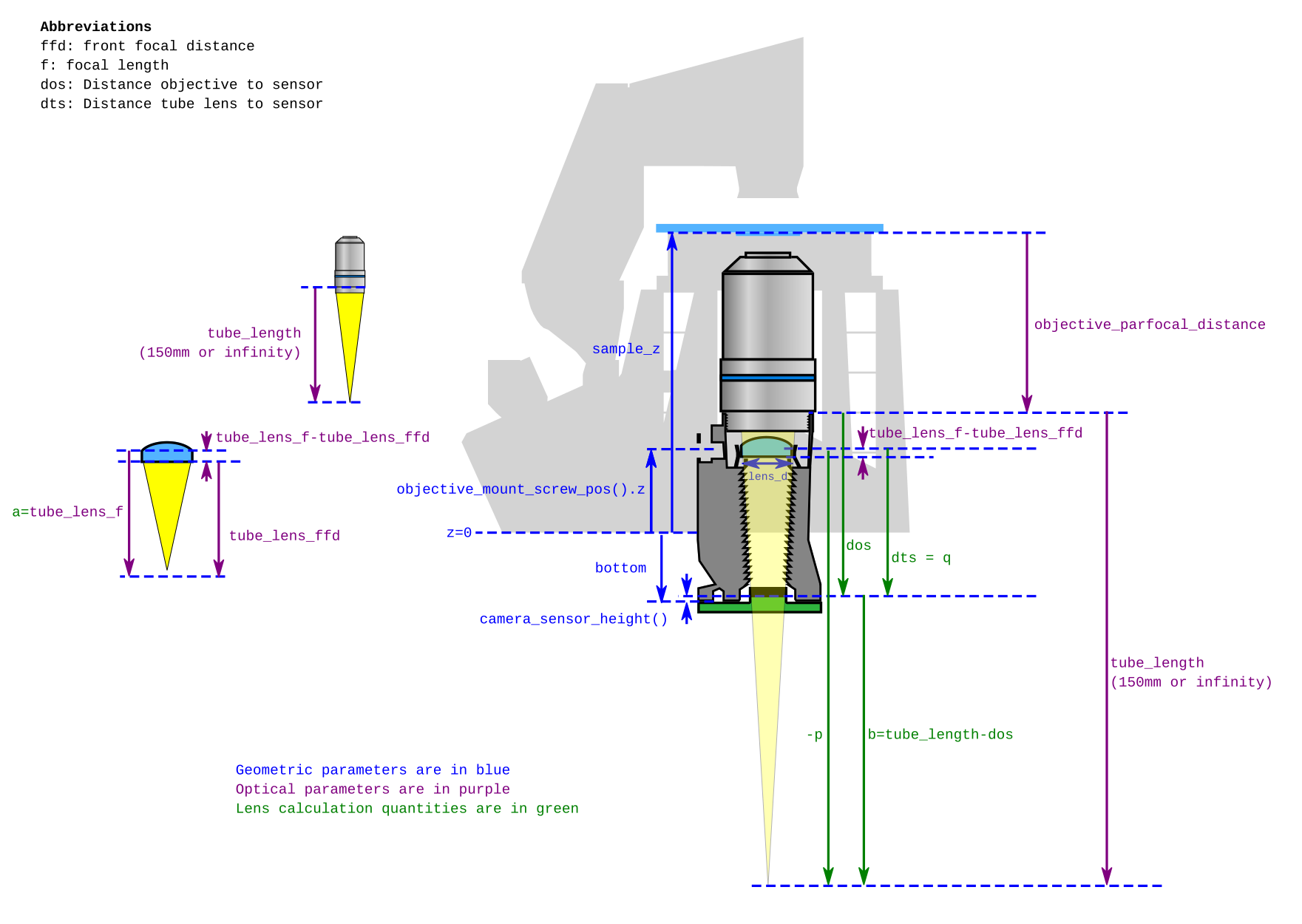Note: that tube length here refers to the back focal length of the objective, not to the mechanical tube length

#### Position of the objective pupil plane

As explained above the pupil plane of the objective is approximately in line with the objective "shoulder" (i.e. where the RMS thread meets the body of the objective). In the code, the position of the objective pupil plane is defined by the position of the sample, sample_z and the "parfocal distance". An unfortunate decision in the past was to do everything for objectives with 35 mm parfocal length, and then simply add in a 10 mm riser on top of the microscope stage if we wanted to use a 45 mm objective. This works fine, but has confused a lot of people in the past - as it means the sample is then 10 mm above sample_z.

• The pupil plane is located at sample_z - objective_parfocal_distance

#### Position of the camera

The actual camera position is set by the relevant camera mount module - but is usually a few mm below the bottom of the main body (i.e. $z<0$). This was originally done to leave clearance for the camera PCB, so it didn't foul the Z mechanism.

There are a few parameters that influence this: the bottom of the camera mount (which is the bottom of the optics module) is called bottom in the code, and is set based on the bottom of the mounting wedge (dt_bottom, set as z=-2 mm to allow for some adjustability), plus space for the camera mount camera_mount_h(), plus an additional space that varies as a function of which optics are used. This additional space is

• 11 mm for a finite conjugate objective and a 50 mm focal length tube lens
• 3 mm for a finite conjugate objective and a 50 mm focal length tube lens
• 23 mm for a infinite conjugate objective and a 50 mm focal length tube lens

This additional space for a sets the distance from the objective to the sensor. For the infinite conjugate objective this ensures there is enough room for the focal length of the lens. For the finite conjugate objective this distance is used in the calculation below. If this number is changed the calculation will ensure the tube lens will still be put in the correct place so that the microscope forms an image, however the demangification from the tube lens will change. Currently the demangification is set so that the image that is formed on the sensor covers approximately the same field of view as would be visible through eyepieces with a 17 mm field number.

The sensor usually protrudes above the PCB, by a few mm in the case of the Raspberry Pi camera due to its plastic housing and foam mount, so:

• the position of the sensor is given by bottom + camera_sensor_height()

We note after code review that camera_sensor_height() may be ignored in some of the calculations. This will have a minor but non-zero impact on where the lens should be.

#### Position of the tube length correction lens

Once we know where the pupil plane is, and where the sensor is, we can calculate the distance from the pupil plane to the sensor.

• The distance from the objective pupil plane to the sensor is
dos = sample_z - objective_parfocal_distance - bottom - camera_sensor_height().
This is denoted $d_{os}$ in equations.

We can then use a thin lens equation (approximating the tube lens as a perfect thin lens) to position the tube length correction lens such that the image is formed on the sensor. The quantity we calculate is the distance from the tube lens (approximated as a thin lens) to the sensor, $d_{ts}$. In order to calculate this we must know the focal length of the tube lens, $f_t$, the back focal length of the objective lens $f_o$, which we call the to tube_length. Applying the thin lens equation to the tube lens, we get:

$$\frac{1}{f_t} = \frac{1}{p} + \frac{1}{q}$$

where $p$ and $q$ are the distances of the object and image planes from the lens. We are effectively imaging a virtual object (the image projected by the objective, to a point beyond the sensor) onto a real image (at the sensor plane), so $p = - f_o + d_{os} - d_{ts}$. $q$ is simply the distance from the tube lens to the sensor, i.e. $q=d_{ts}$. Inserting into the equation above gives

$$\frac{1}{d_{ts}} = \frac{1}{f_t} + \frac{1}{f_o - d_{os} + d_{ts}}$$

multiplying through by $d_{ts}f_t(f_o - d_{os} + d_{ts})$ gives $$f_t(f_o - d_{os} + d_{ts}) = d_{ts}(f_o - d_{os} + d_{ts}) + f_t d_{ts}$$ Multiplying out and then collecting terms for $d_{ts}$ we get $$d_{ts}^2 + (f_o - d_{os})d_{ts} - f_t(f_o - d_{os}) = 0$$ This is a quadratic equation for $d_{ts}$ and can be solved as: $$d_{ts} = \frac{d_{os} - f_o \pm \sqrt{(f_o - d_{os})^2 + 4 f_t(f_o - d_{os})}}{2}$$ If we define $b=f_o - d_{os}$ as done in optics.scad, we get: $$d_{ts} = \frac{-b \pm \sqrt{b^2 + 4 f_t b}}{2}$$ We know $d_{ts}$ should be greater than zero, so we discard the negative root and simplify: $$d_{ts} = \frac{\sqrt{b(4f_t + b)} - b}{2}$$ In optics.scad $d_{ts}$ is denoted as dts and $f_t$ is shortened to a. Note that a and b do not correspond to the standard-form quadratic equation $ax^2 + bx + c = 0$.

Now that we know what d_{ts} should be, we can calculate the position of the tube lens. This is calculated relative to the position of the sensor (bottom + camera_sensor_height()), so the lens ought to be at bottom + camera_sensor_height() + dts. However, the lens is not a "perfect thin lens" and so we need to adjust. This is done by comparing the "front focal distance" to the focal length: see the diagram for the definitions of these two. They should be part of the specifications of the lens you are using, and the ones used in this design are for the ThorLabs AC127-050-A.

• The top of the pillar supporting the lens is
tube_lens_z = bottom + camera_sensor_height() + dts - (tube_lens_f - tube_lens_ffd)

## 3. Implementation details.

### 3.1. Reconfigure code

Reconfigure the optics module code so that the position of all components in the optical train of the optics module are calculated from sample_z. The mounting point should still be calculated from the a z-axis. Any components in an impossible position can throw a warning.

Suggested parameters:

• sample_z - Already used. Sets the sample height above the microscope base.
• objective_parfocal_distance - A parameter in an optics configuration dictionary.
• is_finite_conjugate - A boolean parameter in an optics configuration dictionary. This allows separate calculation for finite and infinite conjugate objectives.
• objective_mechanical_tube_length - A parameter in an optics configuration dictionary. We no longer use the term tube_length to avoid confusion between objective back focal length and mechanical tube length.
• lens_objective_distance - Distance of the tube lens below the objective. - This will be fixed just below the objective to minimise aberrations. Currently for the "standard" configurations of a finite conjugate objective (150 mm tube length) and a 50 mm focal length tube lens this is 8.5588 mm. We will standardise to 8.5 mm.
• lens_sensor_distance - Distance of the tube lens above the camera. - This will be set from the focal length of the tube lens and the the tube length of the objective. Calculation is given below.
• optics_nut_z - The height of the nut trap in the optics mount above the base of the microscope, this is set by the position of the keyhole slot on the z-axtuator.

This is described graphically below. Direction of arrow shows which parts are referenced off another.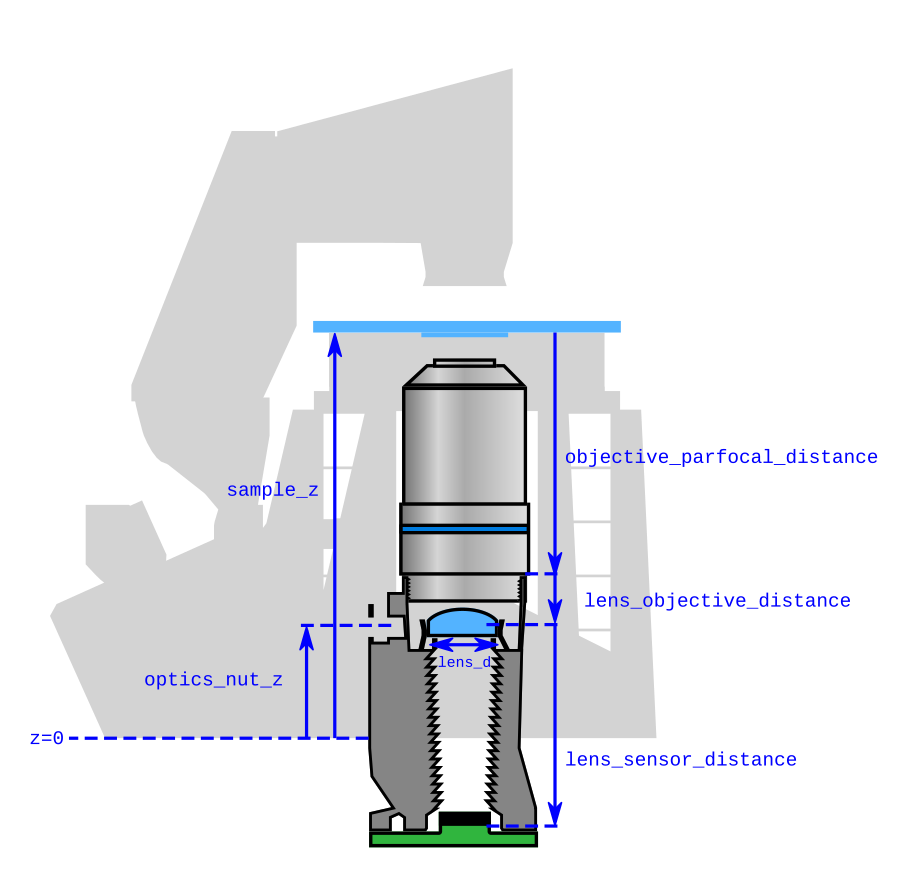A key calculated parameter will be the distance from the tube lens to the primary image plane (or PIP) where the image would have been formed if the tube length was not present. This is the mechanical tube length of the objective, minus the distance between the lens and the objective, minus 10 mm (See section 1.1.). This will become an openscad function:

lens_pip_distance() = objective_mechanical_tube_length - lens_objective_distance - 10

### 3.2. Calculations of lens-sensor distance

Starting again from the thin lens equation: $$\frac{1}{f_t} = \frac{1}{p} + \frac{1}{q}$$

The distances to the image plane, $q$, is lens_sensor_distance. The distance to the virtual object plane $p$ is -lens_pip_distance(), this is negative as it is on the same side of the lens as image plane. As we are now interested in $q$ we can rearrange to: $$q = \frac{f_t p}{p - f_t}$$

After some rearranging we find that

• For infinite conjugate objectives lens_sensor_distance = tube_lens_f
• For finite conjugate objectives lens_sensor_distance = tube_lens_f*(lens_pip_distance())/(lens_pip_distance()+tube_lens_f)

### 3.3. Calculation of desired tube lens

[This is provided for completeness but will be transferred into the documentation]

In this implementation the magnification (or demagnifcation as we are reducing the image size) for a given tube lens is fixed. Rather than tune the value of lens_objective_distance it makes more sense to acquire the correct focal distance lens to give the desired magnification. For the standard optics configuration we will continue to use a Raspberry Pi Camera v2, and a 50 mm focal length tube lens. For those using a custom camera the documentation should be clear on

• The desired magnification for the sensor size
• The desired focal length of the tube lens
• The effective field number for the a given lens

A traditional microscope will form an image that can be viewed with an eyepiece. The eyepiece will have an aperture at this image plane, this will set the field of view. This aperture size is called the field number (FN) and is quoted in millimeters. The field number can range from about 14-26 mm depending on the application. We assume 17 mm to be a standard field number. The sensor of most cameras is much smaller than this. The desired magnification should match the field of view of the eyepiece to the field of view of the camera sensor: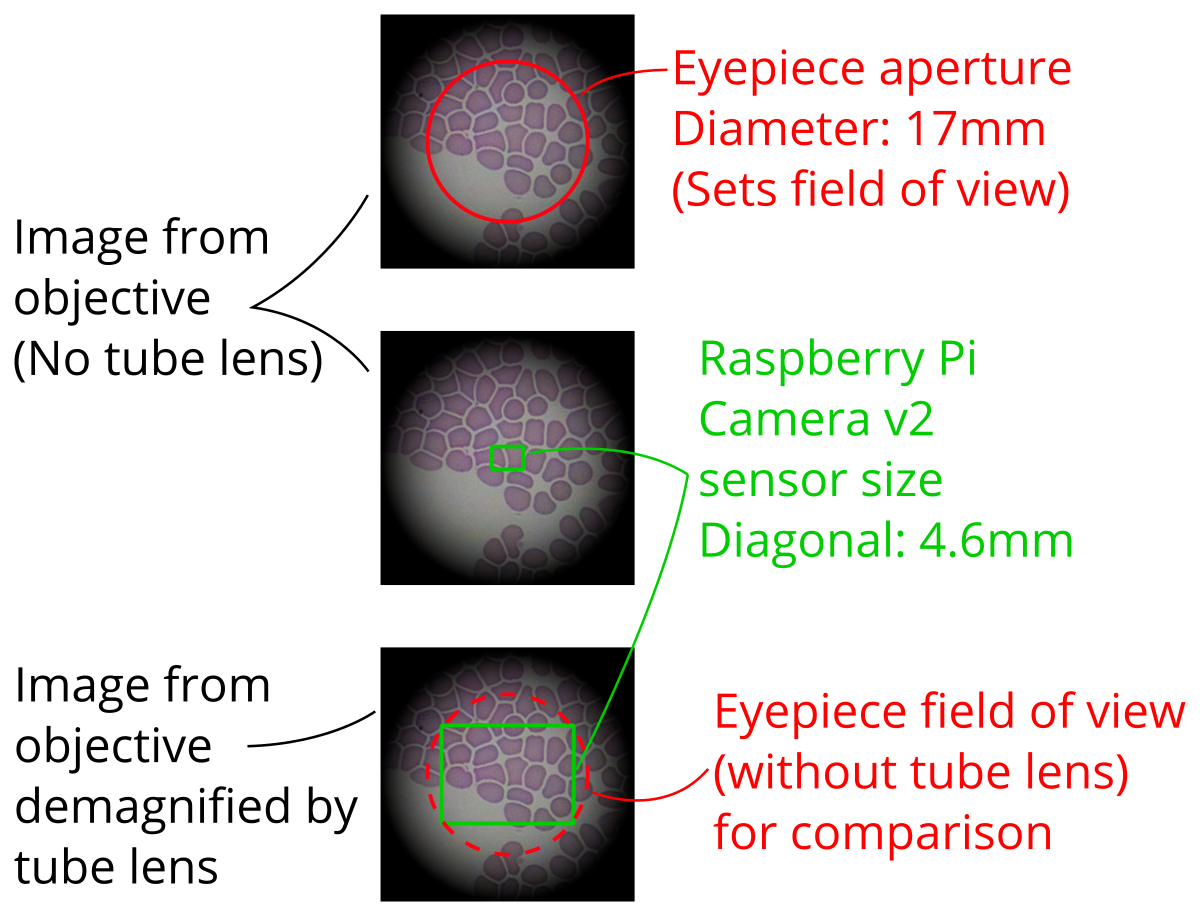We calculate the desired magnification as

$$\text{Magnification} = \frac{\text{Diagonal sensor size}}{\text{Field number}}$$ In the case of the Raspberry Pi Camera v2, the diagonal sensor size is 4.6 mm so the magnification is 4.6/17 = 0.27.

The magnification, $M$, is equal to $$M = \frac{q}{p}$$ If we insert this the thin lens equation above we get can rearrange this to give $$f_t = \frac{M}{M-1}p$$ From above we know that $p$ is calculated as -lens_pip_distance(). This is distance from the lens to the objective (now set to 8.5 mm) minus the back focal length (150 mm). Therefore, for the Raspberry Pi Camera v2 the we calculate the optimal focal length for the tube lens as $$f_t = \frac{0.27}{0.27-1}(8.5 \,\mathrm{mm} - 150 \,\mathrm{mm}) = 52.3 \,\mathrm{mm}$$

We then choose 50 mm as the focal length as it is an easy to purchase focal length. This has a slight effect on the magnification. By rearranging the above we calculate the magnification for this lens as $$M = \frac{f}{f-p}$$ For the Raspberry Pi Camera v2 and a 50 mm focal length tube lens $$M = \frac{50 \,\mathrm{mm}}{50 \,\mathrm{mm} - 8.5 \,\mathrm{mm} + 150 \,\mathrm{mm}} = 0.261$$

We can now calculate the effective field number of the diagonal of the Raspberry Pi Camera v2 sensor as $$\text{Effective field number} = \frac{4.6 \,\mathrm{mm}}{0.261} = 17.6 \,\mathrm{mm}$$ This falls well within the range of standard eyepiece field numbers (14-26 mm).